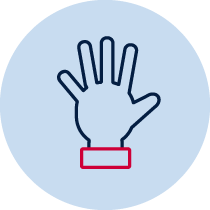# Whole number – creating 20

Students develop confidence with number sequences to 100 by ones from any starting point.Practical Resource required

## Number and algebra – whole number

• describes mathematical situations and methods using everyday and some mathematical language, actions, materials, diagrams and symbols MA1-1WM
• uses objects, diagrams and technology to explore mathematical problems MA1-2WM
• supports conclusions by explaining or demonstrating how answers were obtained MA1-3WM
• applies place value, informally, to count, order, read and represent two- and three-digit numbers MA1-4NA

## Content

Develop confidence with number sequences to 100 by ones from any starting point (ACMNA012)

## National Numeracy Learning Progression mapping to the NSW mathematics syllabus

When working towards the outcome MA1-4NA the sub-elements (and levels) of Quantifying numbers (QuN6-QuN8) and Understanding money (UnM3-UnM4) describe observable behaviours that can aid teachers in making evidence based decisions about student development and future learning.

## Materials

• 1-20 numeral cards

## Teacher instructions

The purpose of this task is to gauge students’ understanding of whole number concepts such as:

• identify the number before a given two-digit number
• identify the number after a given two-digit number
• describe a number before as 'one less than' and the number after as 'one more than' a given number

Students are presented with shuffled numbers cards with the numbers 1 to 20. Instruct students to place the cards face down in two rows of 10.

Students turn over the first card in the top right hand corner and read it aloud. They place the card, face up, where it should go if the cards were in order. They explain the reason for their placement. Students then pick up the card replaced and continue to arrange the numbers in order. For example, if the first card they turn up is the number 5, they say ‘5’, place it where the 5 should go and explain the reason why they placed it in that position. The student takes the card replaced and repeats the process.

## Student instructions

Place the cards face down in two rows of 10.

Turn over the first card in the top right hand corner.

Read the number displayed on the card.

Place this card, face up, where it should go if the numbers were in order.

Explain why you placed the card in that position.

Pick up the card that it replaces and find out where it should be placed.

Continue till all the cards are flipped over.

If a card is in the right place when turned over, explain how you know. Then select another face down card and continue the task.

## Possible areas for further exploration

Is the student reliant on a particular strategy to order the numbers?

What reason/s does the student provide?

## Where to next?

The task could be repeated using:

• a focus on the backward sequence
• a different range of numbers